arrow_back_iosQuestions
help

# Our price for one hour is \$100, but from the third hour the price becomes \$80. How to configure it?

check_circle
Let's say you already have a resource with a rule of work. For example, a rental for \$100 / hour for each item. And only 10 places are available.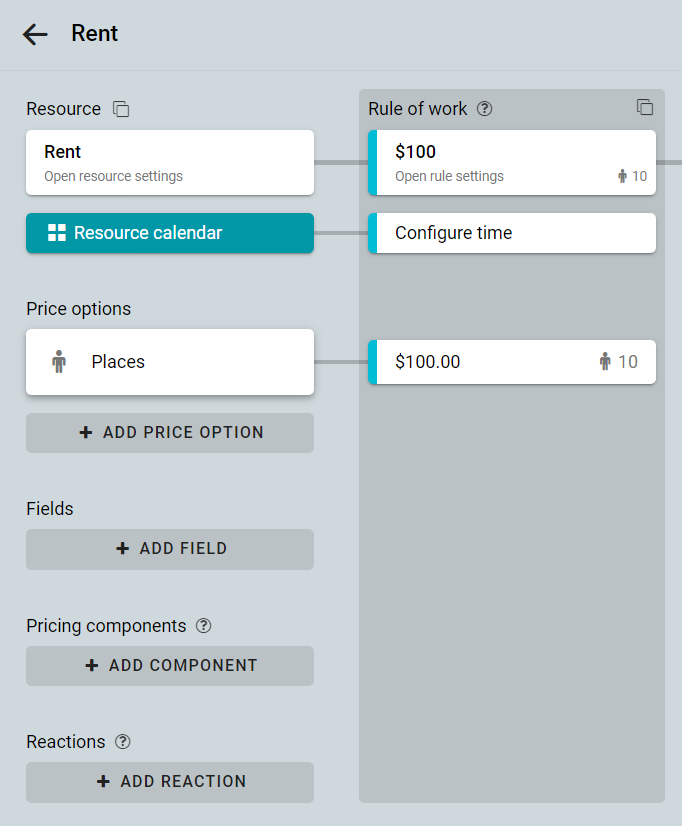To change the price of the third and subsequent hours, you can use one of three solutions.

## Discount for long-term booking

You can add a discount for reservations that last 3 hours or more.
Create a pricing component.
Enter the name of the component. Click on the Insert parameter button. This way you can insert parameters for duration — number of hours [STEPS] and for shares — number of places [P1].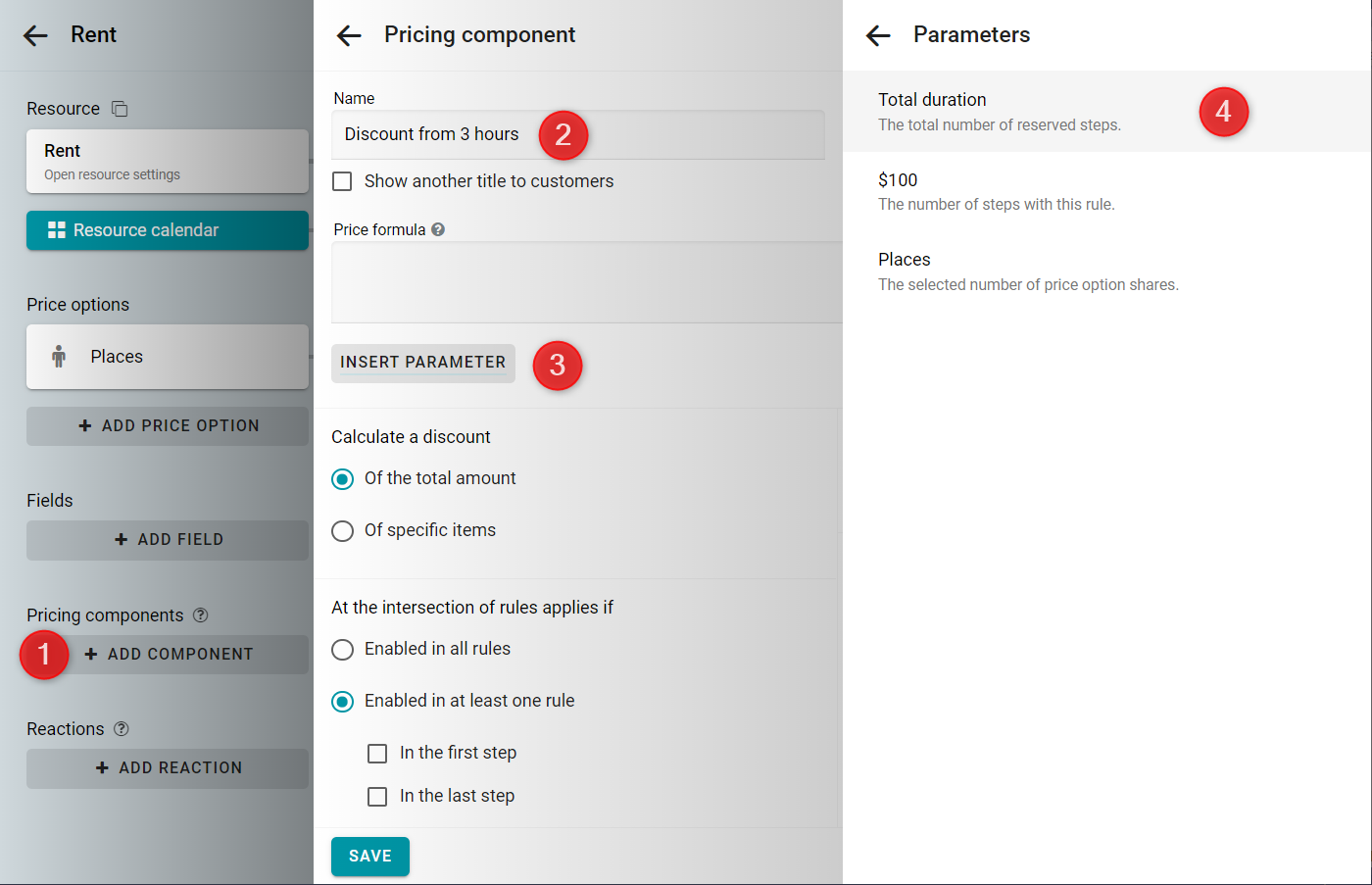Enter the formula. The discount of \$20 is multiplied by the number of hours over two. And we multiply all this by the number of reserved places.
-20 * ([STEPS]-2) * [P1]
After that click on the Add condition button.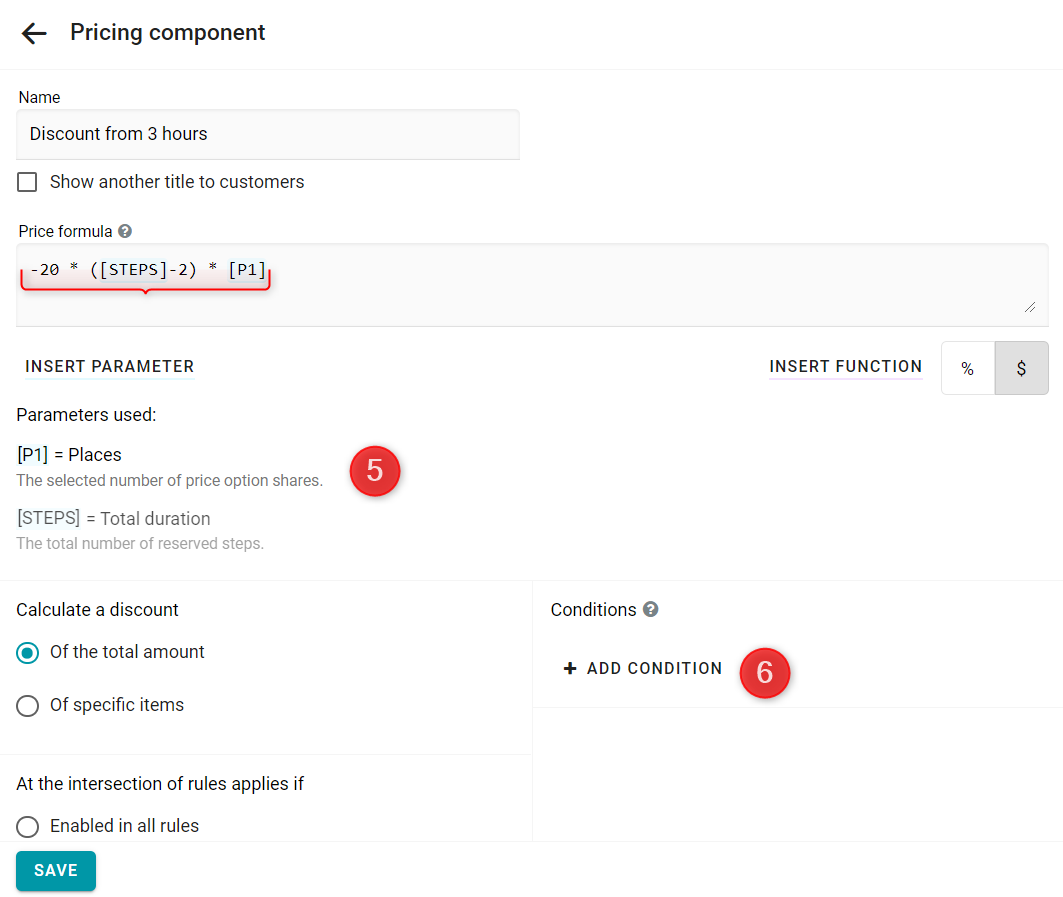Set the minimum steps — 3. In this case, when booking one or two periods, the discount will not be applied. Save it.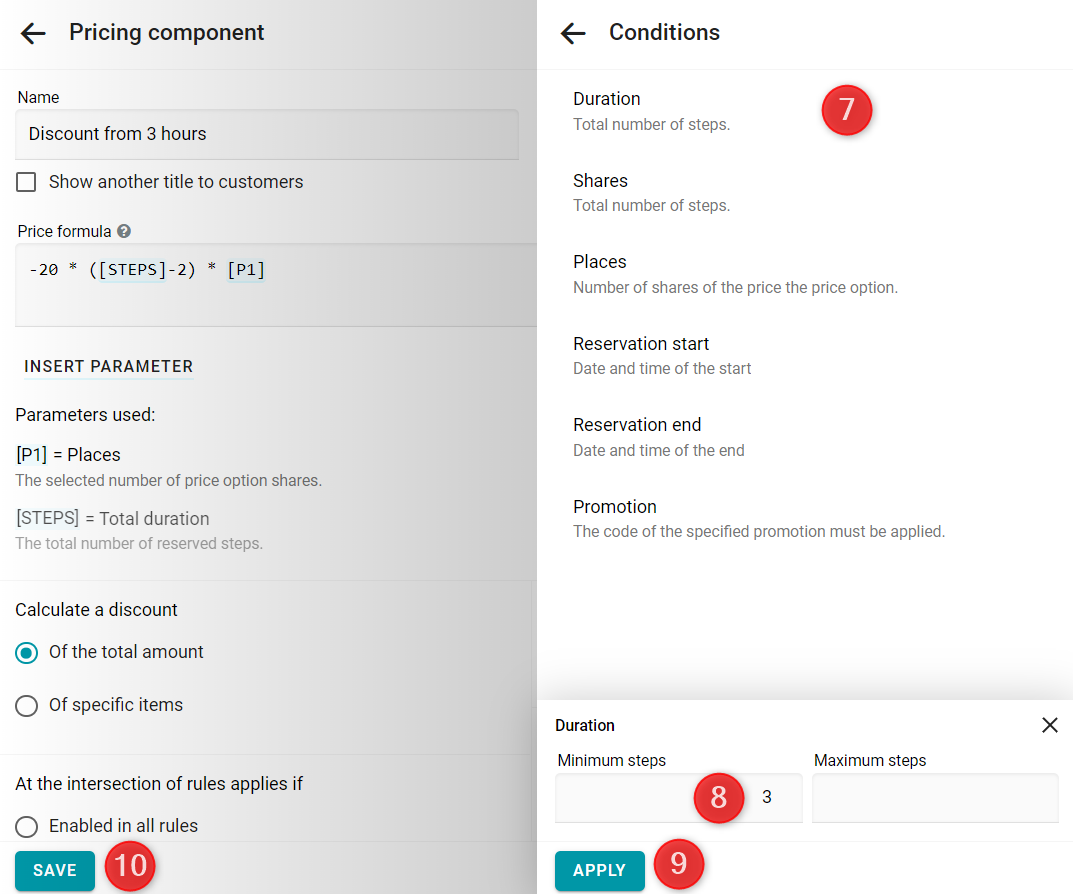Now let's check the result. We will book two seats for 5 hours.
-20 * (5-2) * 2 = \$120.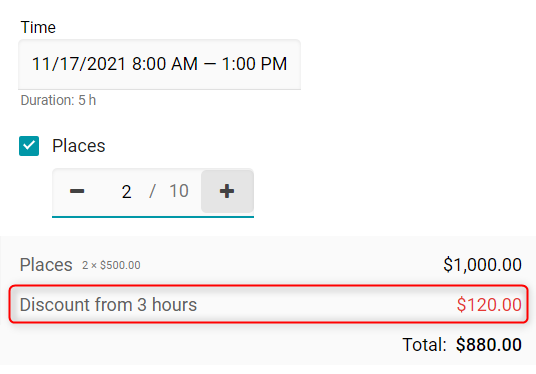## Extra charge for short-term bookings

You can go on the opposite way. Add a surcharge of \$20 per hour for bookings shorter than three hours. To do this, first set the price of the hour — \$80. Then create a pricing component.
Enter the name of the component. Insert parameters for duration — number of hours [STEPS] and for shares — number of places [P1].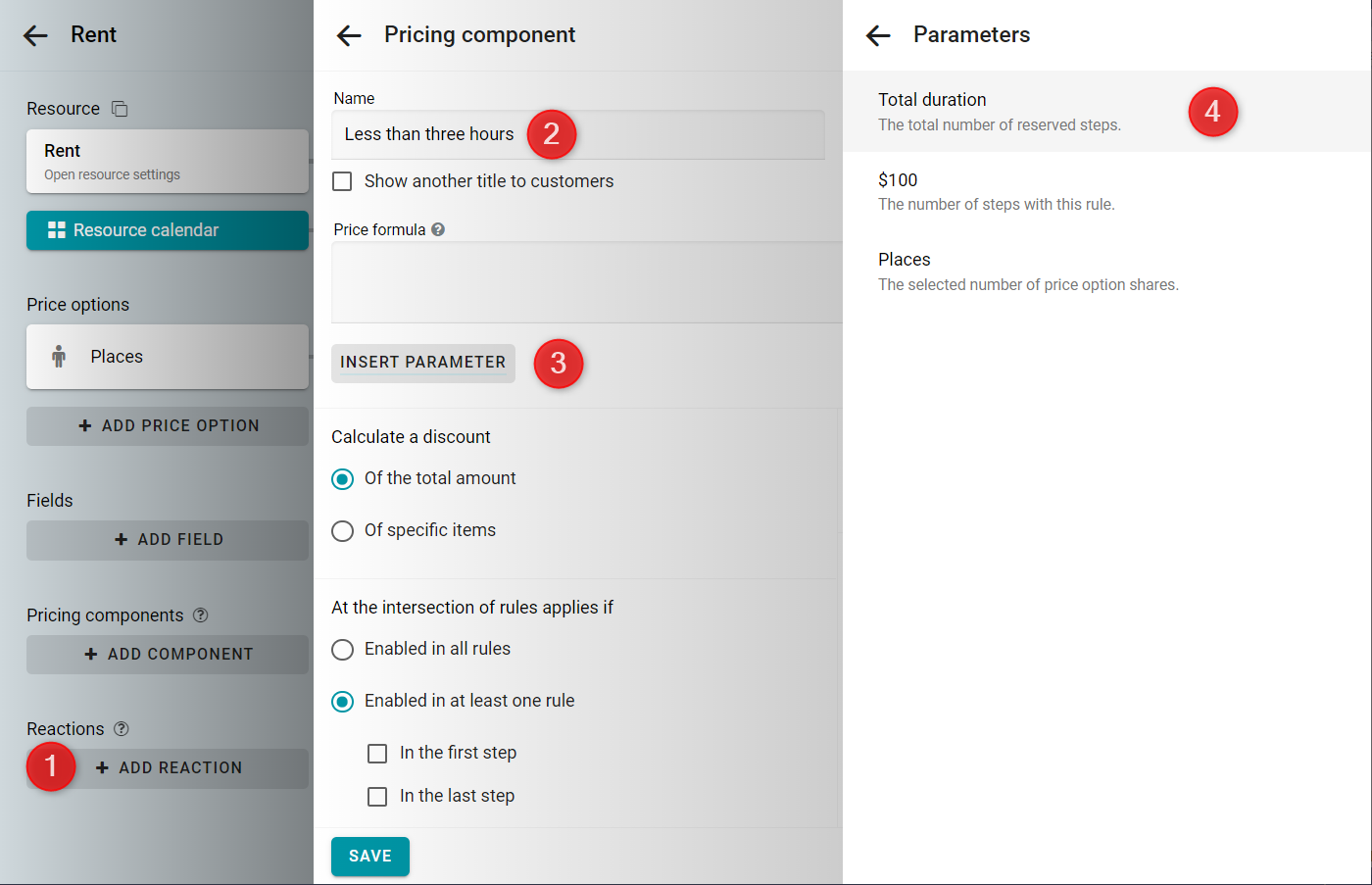Enter the formula. The charge of \$20 is multiplied by the number of hours. And all this is multiplied by the number of seats reserved.
20 * [STEPS] * [P1]
After that click on the Add condition button.Set the maximum steps — 2. Then the discount will only be applied for bookings of one or two periods. Save.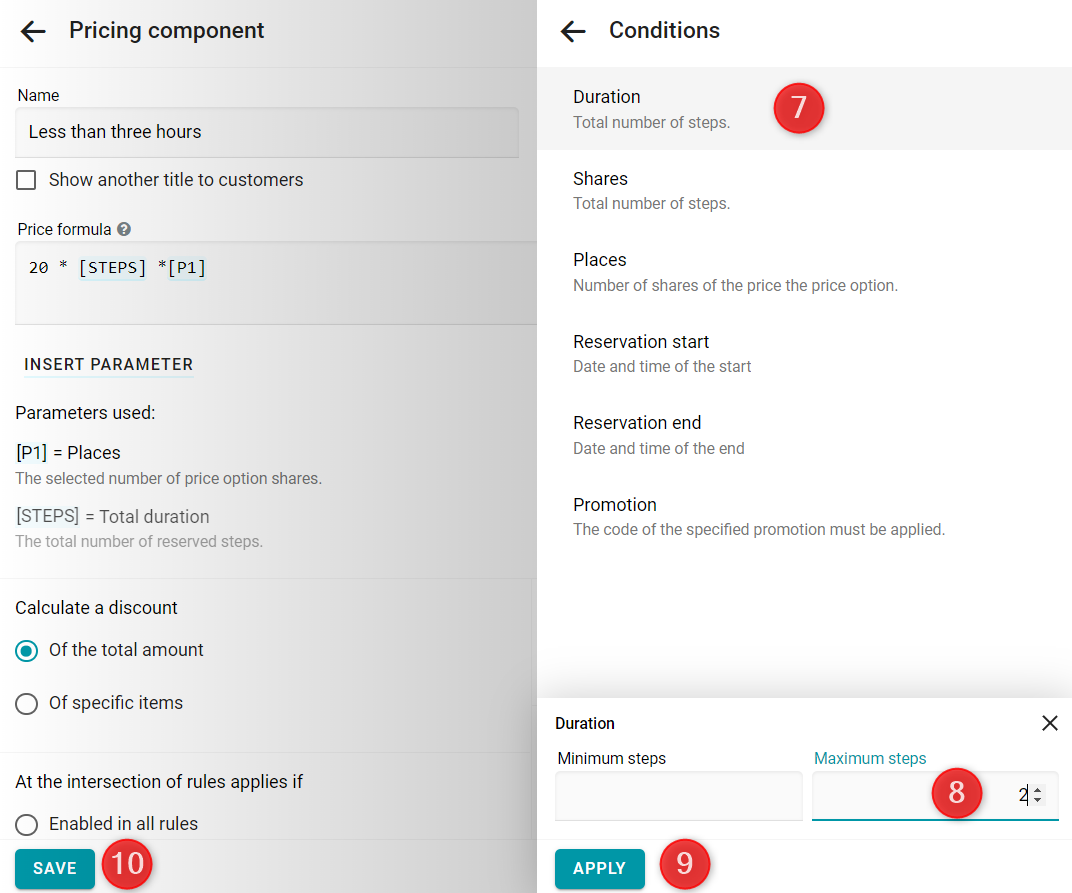Check the results. We'll book 3 seats for 2 hours.
20 * 2 * 3 = \$120.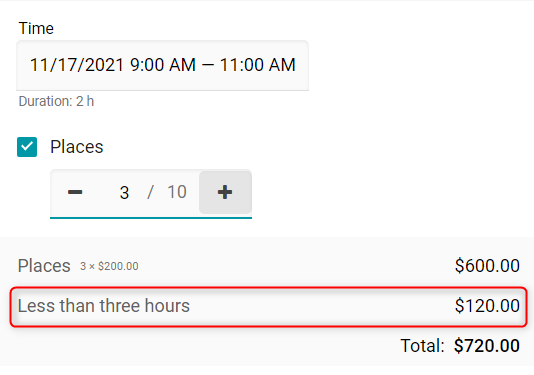## Price by the pricing component

If you don't want to split the cost into parts, calculate it via the single pricing component. To do this, set the price of the price option — \$0. Create the pricing component.
Enter the name of the component. Insert parameters for duration — number of hours [STEPS] and for shares — number of places [P1].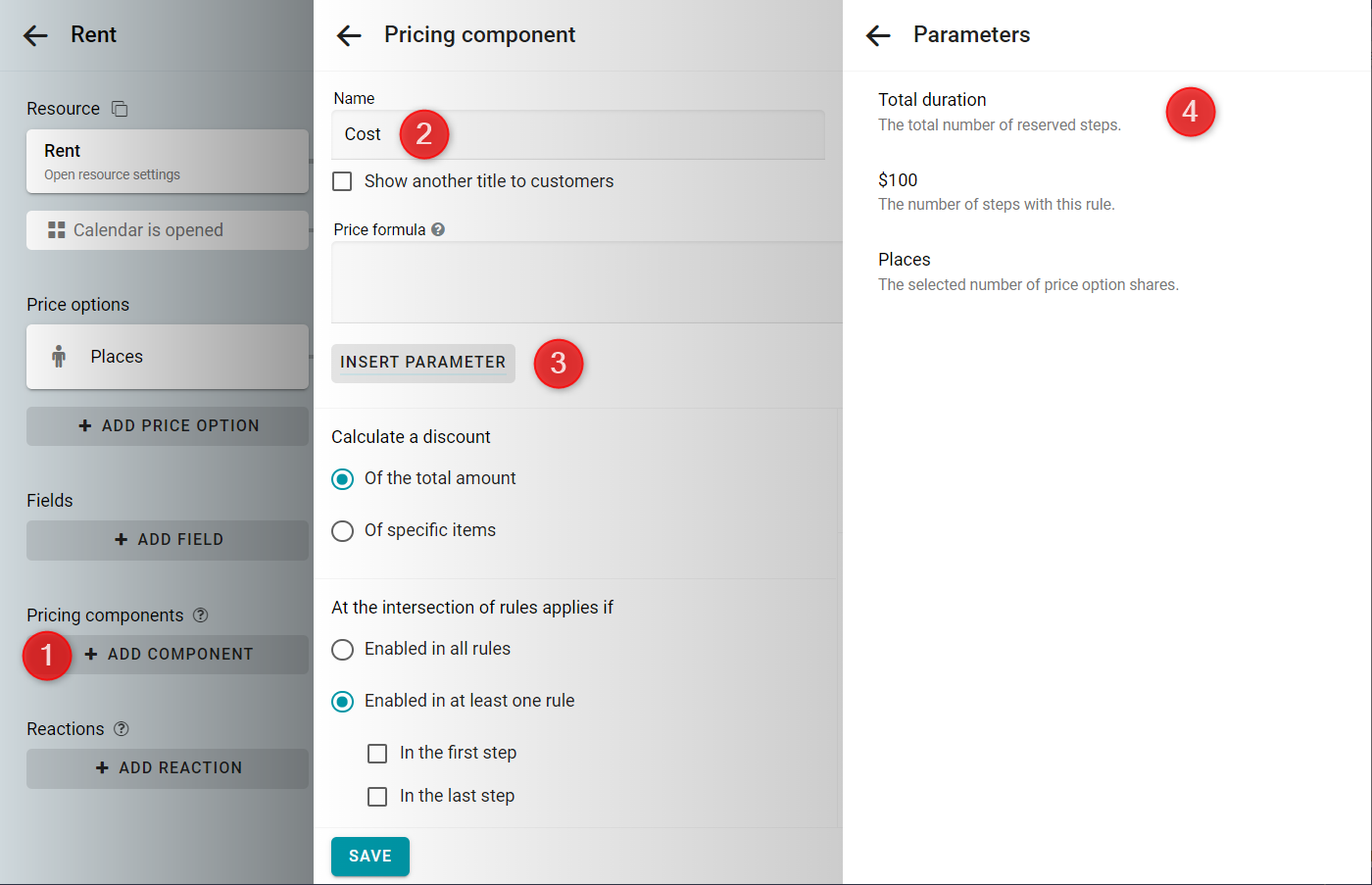Enter the formula. The price of \$100 is multiplied by the number of hours. Subtract the discount of \$20 multiplied by the number of hours over two. And all this is multiplied by the number of reserved places.
In formula, use the function called Max. It returns the biggest of two numbers. Thus, the expression [STEPS]-2 cannot become less than zero. In order to add a function click on the Insert function button and pick Max from the list of functions.
(100 * [STEPS] - 20 * Max(0,[STEPS]-2)) * [P1]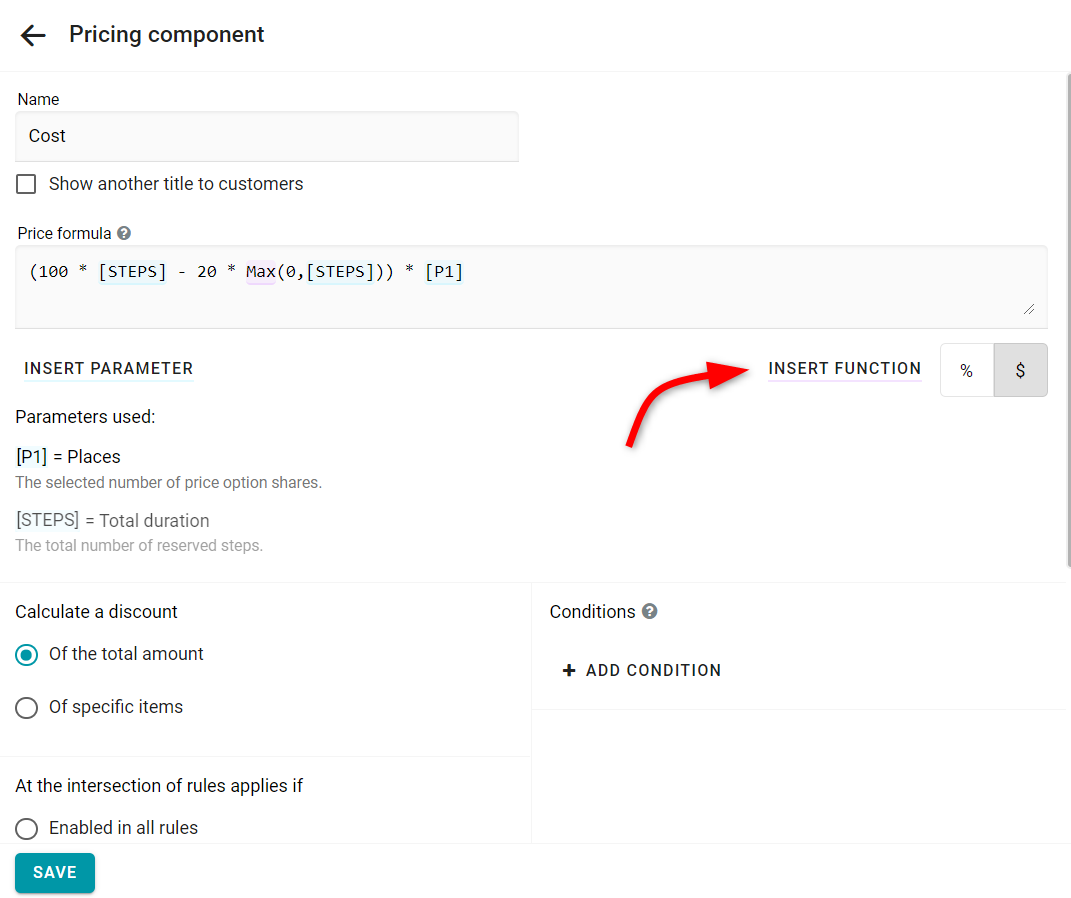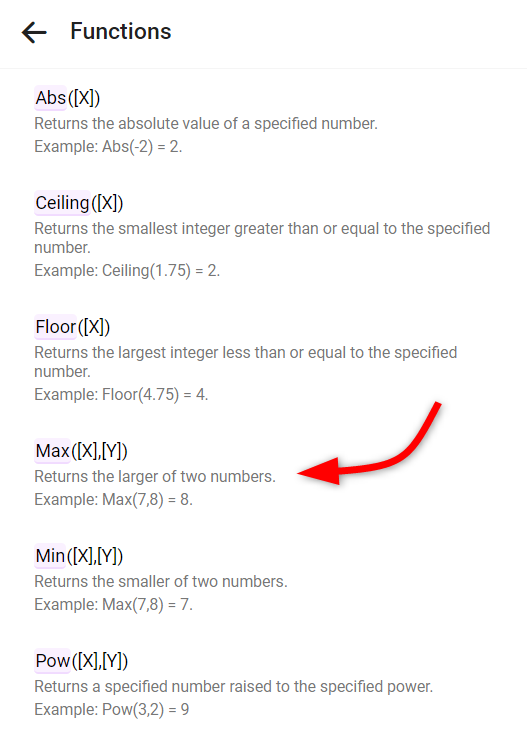Let's check the result. We will reserve two seats for 4 hours.
(100 * 4 - 20 * (4-2)) * 2 = \$720.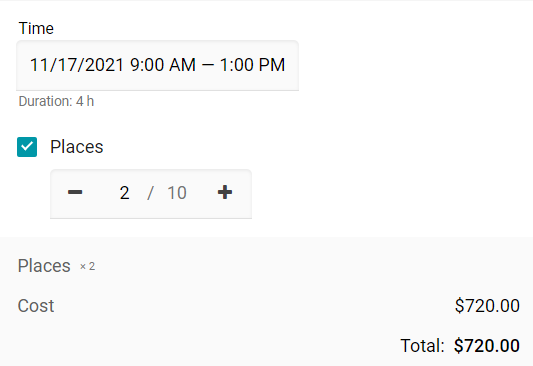See this example in action: